Скачать презентацию 10 c Machine Learning Symbol-based 10 0 Introduction

e91c2d7a74574ca6895144e7e00dabf6.ppt

• Количество слайдов: 35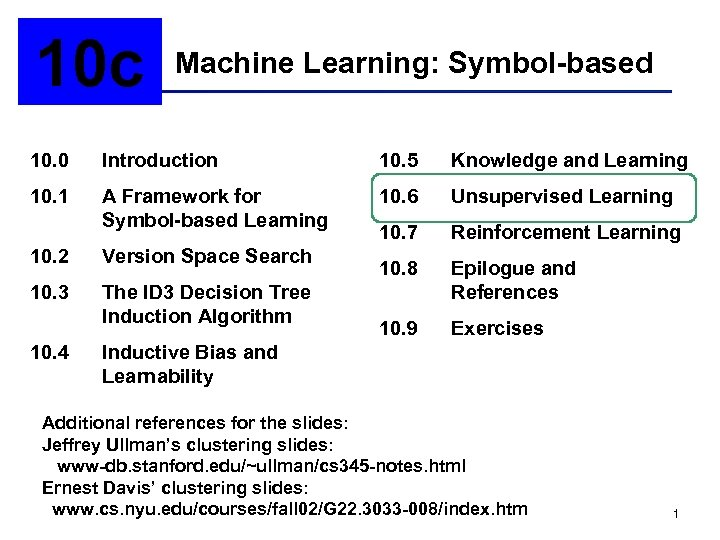10 c Machine Learning: Symbol-based 10. 0 Introduction 10. 5 Knowledge and Learning 10. 1 A Framework for Symbol-based Learning 10. 6 Unsupervised Learning 10. 7 Reinforcement Learning 10. 8 Epilogue and References 10. 9 Exercises 10. 2 Version Space Search 10. 3 The ID 3 Decision Tree Induction Algorithm 10. 4 Inductive Bias and Learnability Additional references for the slides: Jeffrey Ullman’s clustering slides: www-db. stanford. edu/~ullman/cs 345 -notes. html Ernest Davis’ clustering slides: www. cs. nyu. edu/courses/fall 02/G 22. 3033 -008/index. htm 1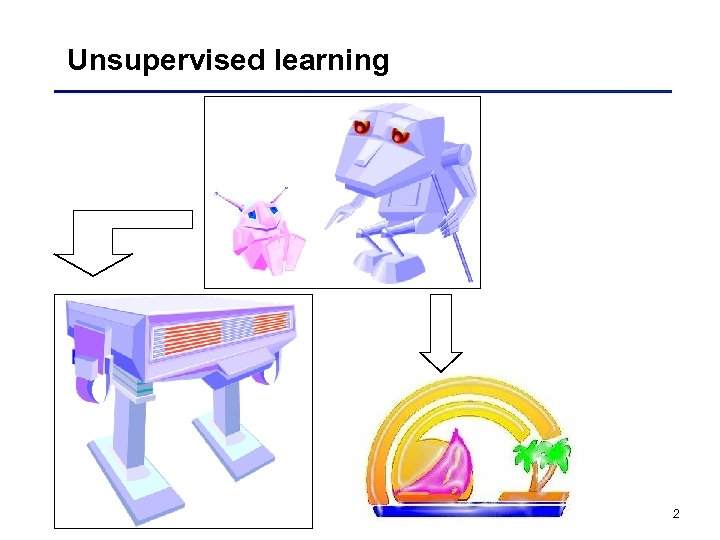Unsupervised learning 2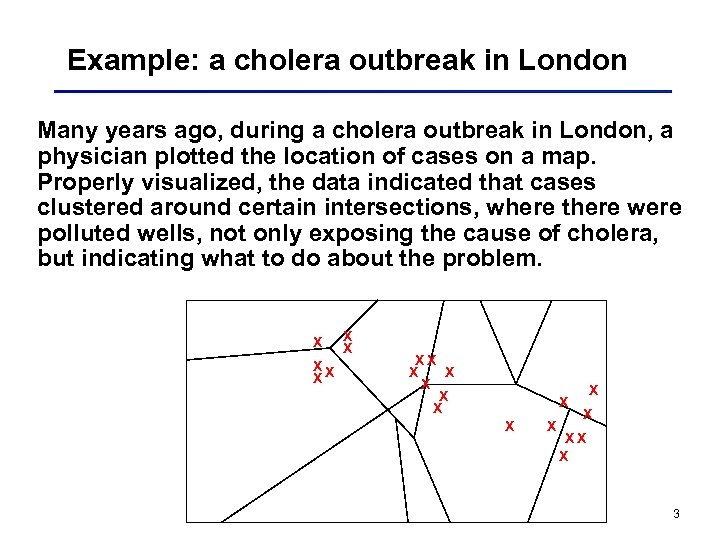Example: a cholera outbreak in London Many years ago, during a cholera outbreak in London, a physician plotted the location of cases on a map. Properly visualized, the data indicated that cases clustered around certain intersections, where there were polluted wells, not only exposing the cause of cholera, but indicating what to do about the problem. X XX X X XX X 3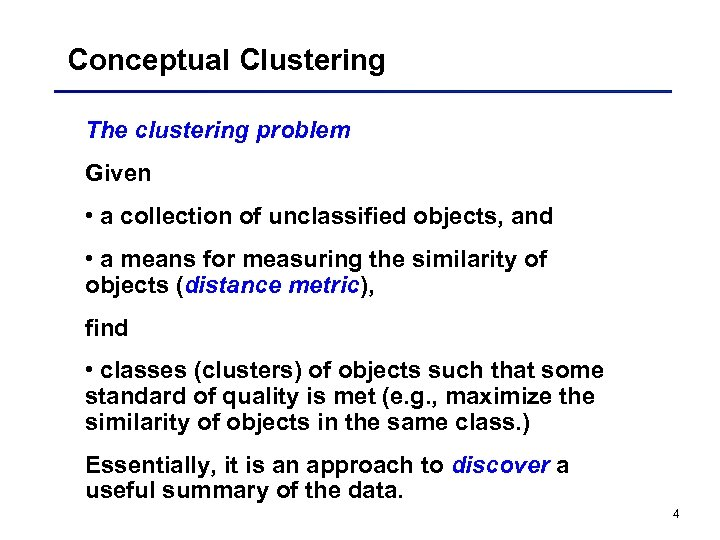Conceptual Clustering The clustering problem Given • a collection of unclassified objects, and • a means for measuring the similarity of objects (distance metric), find • classes (clusters) of objects such that some standard of quality is met (e. g. , maximize the similarity of objects in the same class. ) Essentially, it is an approach to discover a useful summary of the data. 4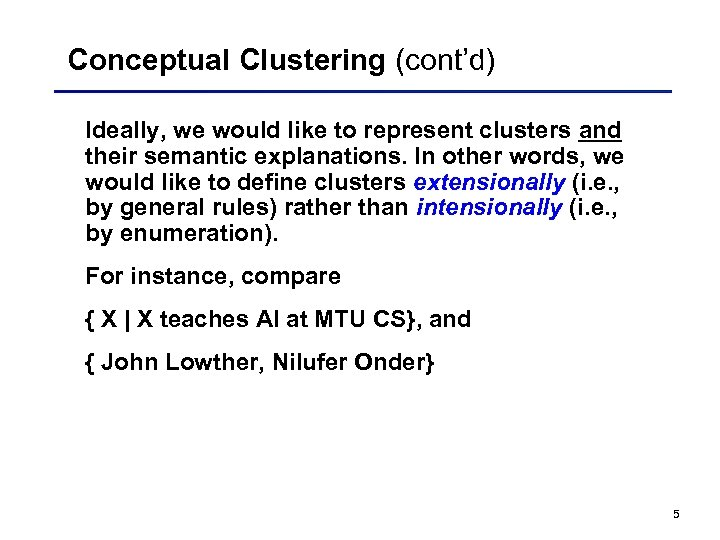Conceptual Clustering (cont’d) Ideally, we would like to represent clusters and their semantic explanations. In other words, we would like to define clusters extensionally (i. e. , by general rules) rather than intensionally (i. e. , by enumeration). For instance, compare { X | X teaches AI at MTU CS}, and { John Lowther, Nilufer Onder} 5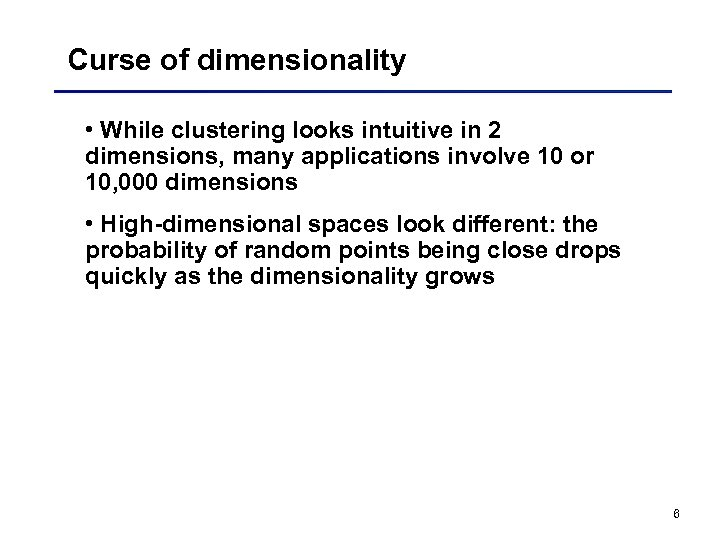Curse of dimensionality • While clustering looks intuitive in 2 dimensions, many applications involve 10 or 10, 000 dimensions • High-dimensional spaces look different: the probability of random points being close drops quickly as the dimensionality grows 6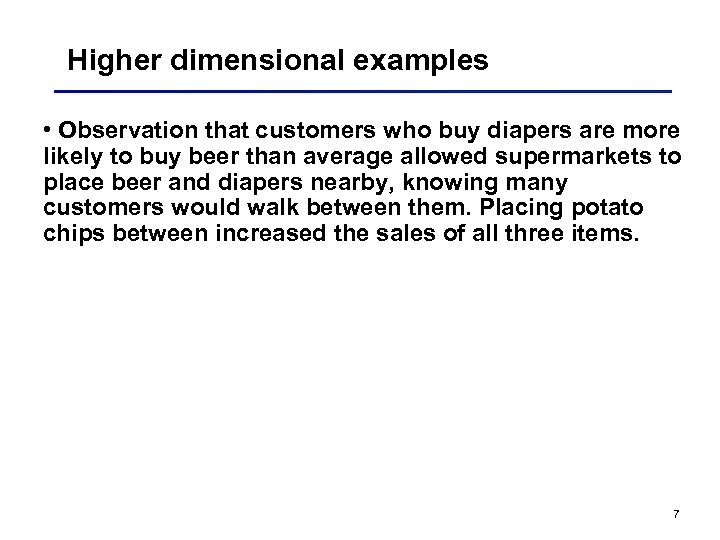Higher dimensional examples • Observation that customers who buy diapers are more likely to buy beer than average allowed supermarkets to place beer and diapers nearby, knowing many customers would walk between them. Placing potato chips between increased the sales of all three items. 7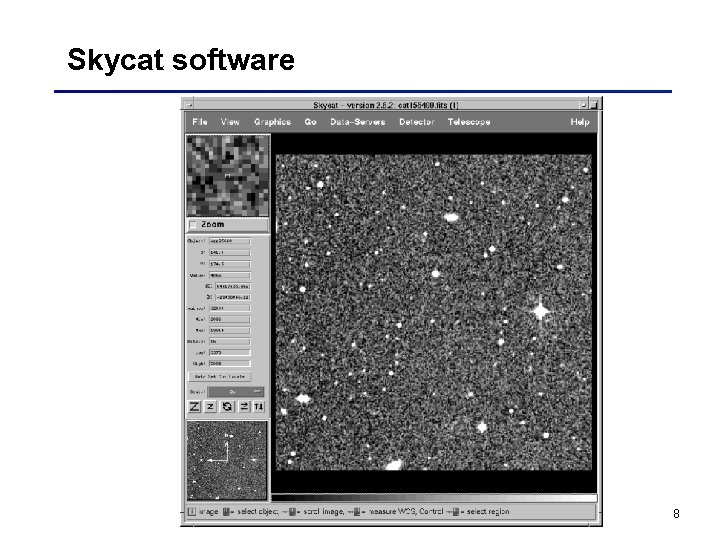Skycat software 8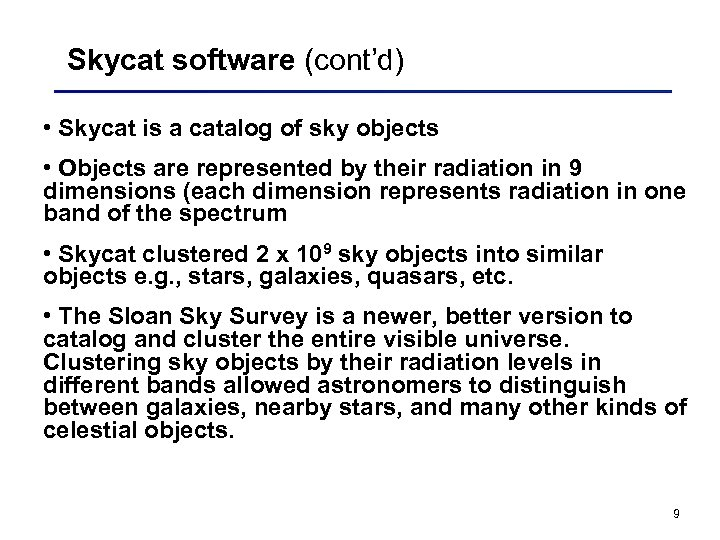Skycat software (cont’d) • Skycat is a catalog of sky objects • Objects are represented by their radiation in 9 dimensions (each dimension represents radiation in one band of the spectrum • Skycat clustered 2 x 109 sky objects into similar objects e. g. , stars, galaxies, quasars, etc. • The Sloan Sky Survey is a newer, better version to catalog and cluster the entire visible universe. Clustering sky objects by their radiation levels in different bands allowed astronomers to distinguish between galaxies, nearby stars, and many other kinds of celestial objects. 9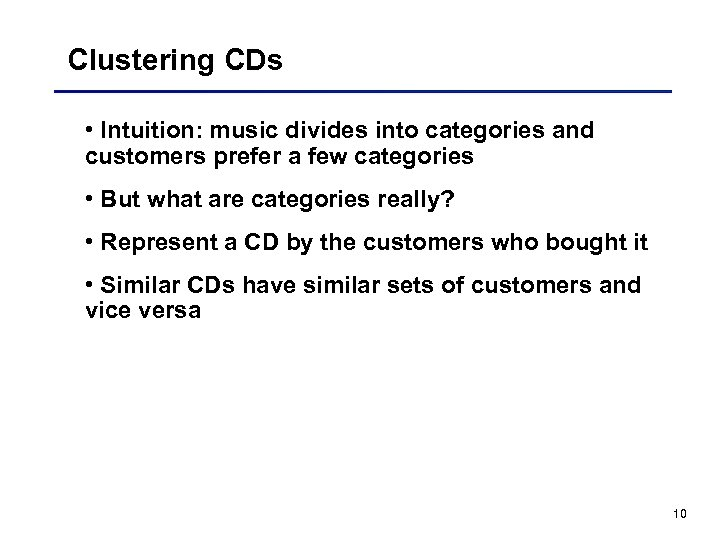Clustering CDs • Intuition: music divides into categories and customers prefer a few categories • But what are categories really? • Represent a CD by the customers who bought it • Similar CDs have similar sets of customers and vice versa 10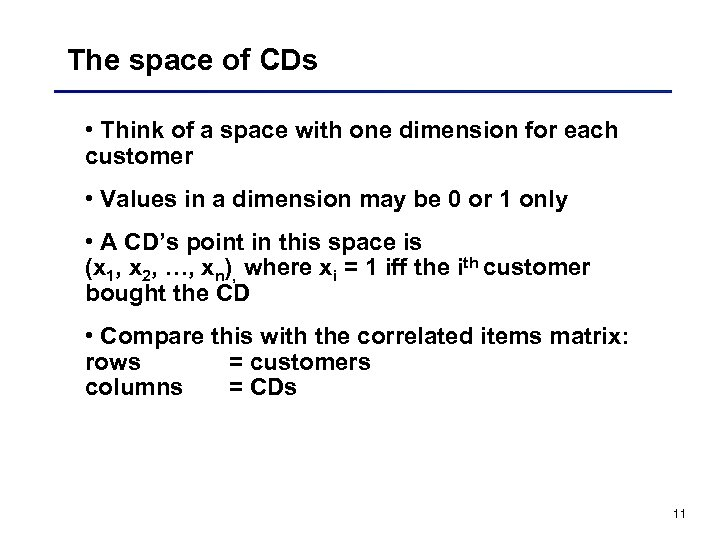The space of CDs • Think of a space with one dimension for each customer • Values in a dimension may be 0 or 1 only • A CD’s point in this space is (x 1, x 2, …, xn), where xi = 1 iff the ith customer bought the CD • Compare this with the correlated items matrix: rows = customers columns = CDs 11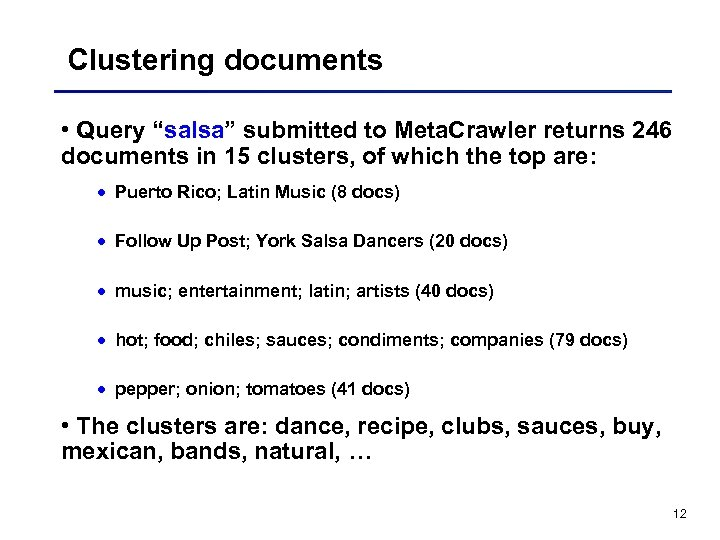Clustering documents • Query “salsa” submitted to Meta. Crawler returns 246 documents in 15 clusters, of which the top are: · Puerto Rico; Latin Music (8 docs) · Follow Up Post; York Salsa Dancers (20 docs) · music; entertainment; latin; artists (40 docs) · hot; food; chiles; sauces; condiments; companies (79 docs) · pepper; onion; tomatoes (41 docs) • The clusters are: dance, recipe, clubs, sauces, buy, mexican, bands, natural, … 12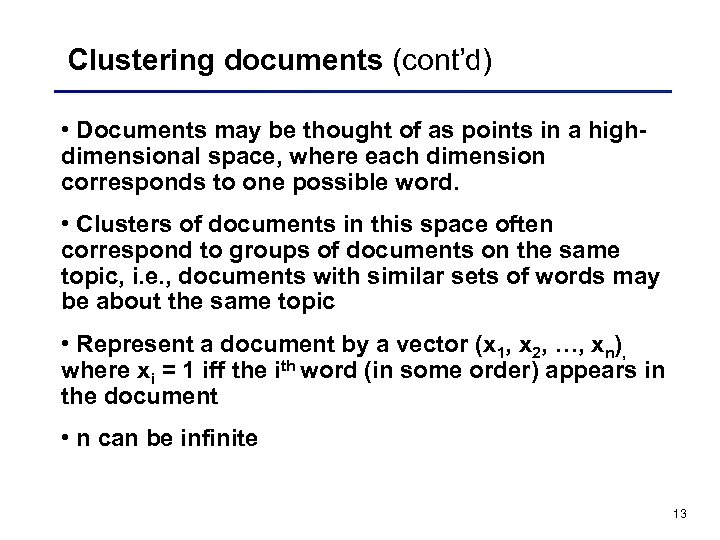Clustering documents (cont’d) • Documents may be thought of as points in a highdimensional space, where each dimension corresponds to one possible word. • Clusters of documents in this space often correspond to groups of documents on the same topic, i. e. , documents with similar sets of words may be about the same topic • Represent a document by a vector (x 1, x 2, …, xn), where xi = 1 iff the ith word (in some order) appears in the document • n can be infinite 13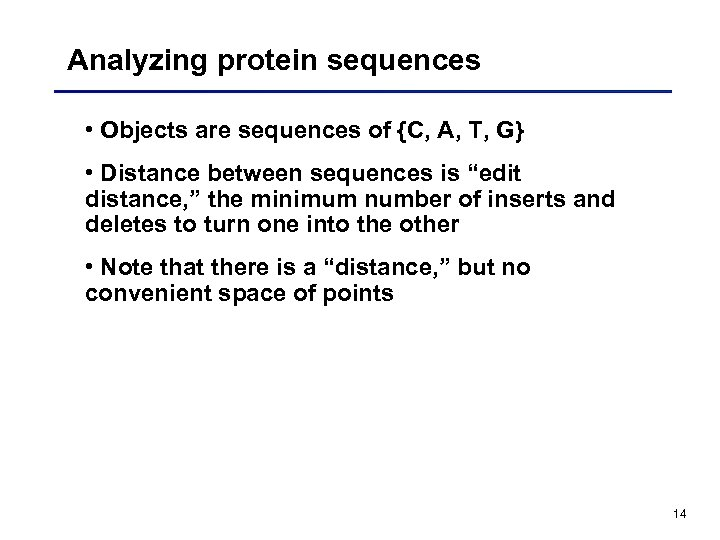Analyzing protein sequences • Objects are sequences of {C, A, T, G} • Distance between sequences is “edit distance, ” the minimum number of inserts and deletes to turn one into the other • Note that there is a “distance, ” but no convenient space of points 14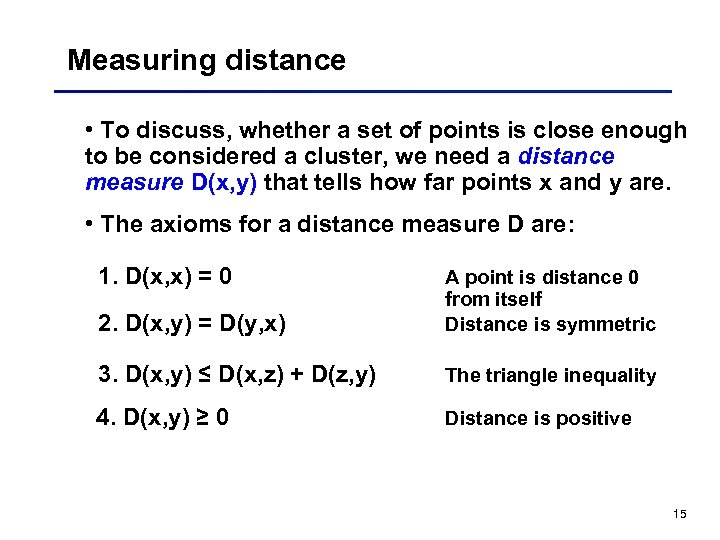Measuring distance • To discuss, whether a set of points is close enough to be considered a cluster, we need a distance measure D(x, y) that tells how far points x and y are. • The axioms for a distance measure D are: 1. D(x, x) = 0 2. D(x, y) = D(y, x) A point is distance 0 from itself Distance is symmetric 3. D(x, y) ≤ D(x, z) + D(z, y) The triangle inequality 4. D(x, y) ≥ 0 Distance is positive 15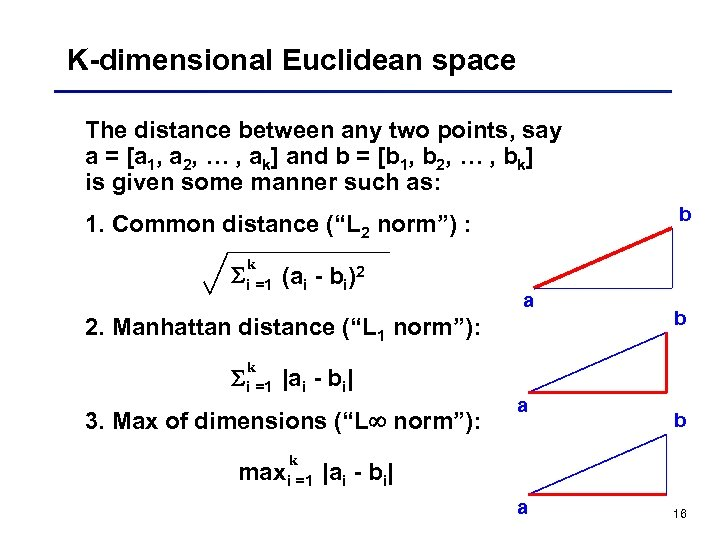K-dimensional Euclidean space The distance between any two points, say a = [a 1, a 2, … , ak] and b = [b 1, b 2, … , bk] is given some manner such as: b 1. Common distance (“L 2 norm”) : k i =1 (ai - bi)2 a 2. Manhattan distance (“L 1 norm”): k i =1 |ai - bi| 3. Max of dimensions (“L norm”): k maxi =1 a b b |ai - bi| a 16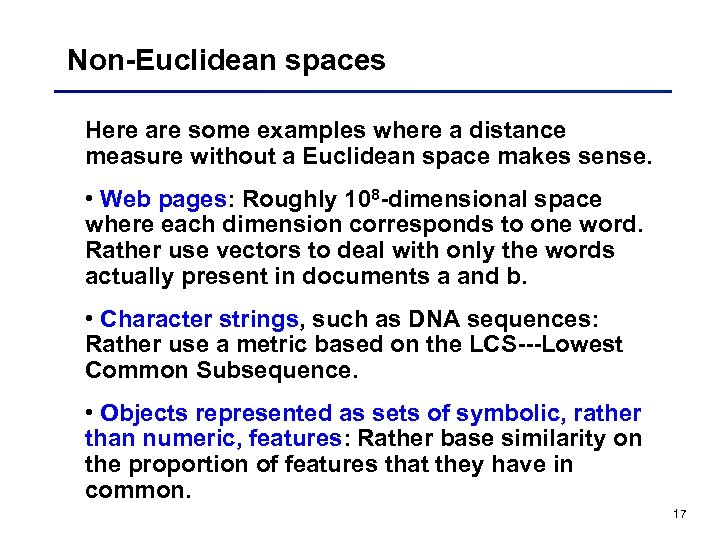Non-Euclidean spaces Here are some examples where a distance measure without a Euclidean space makes sense. • Web pages: Roughly 108 -dimensional space where each dimension corresponds to one word. Rather use vectors to deal with only the words actually present in documents a and b. • Character strings, such as DNA sequences: Rather use a metric based on the LCS---Lowest Common Subsequence. • Objects represented as sets of symbolic, rather than numeric, features: Rather base similarity on the proportion of features that they have in common. 17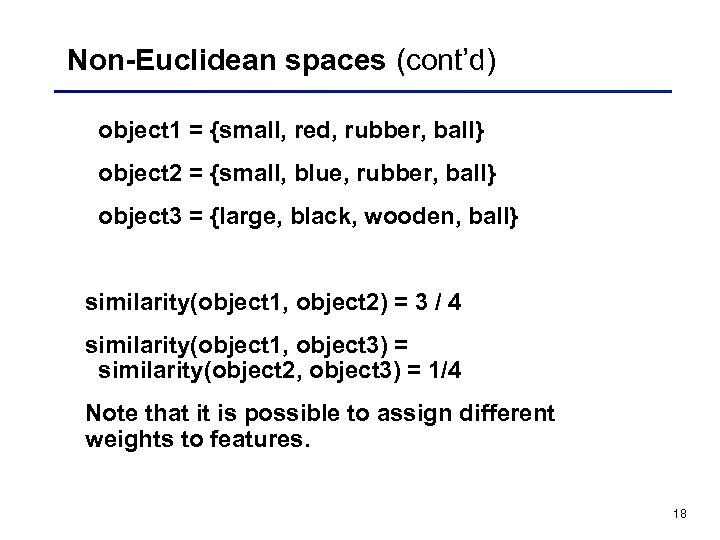Non-Euclidean spaces (cont’d) object 1 = {small, red, rubber, ball} object 2 = {small, blue, rubber, ball} object 3 = {large, black, wooden, ball} similarity(object 1, object 2) = 3 / 4 similarity(object 1, object 3) = similarity(object 2, object 3) = 1/4 Note that it is possible to assign different weights to features. 18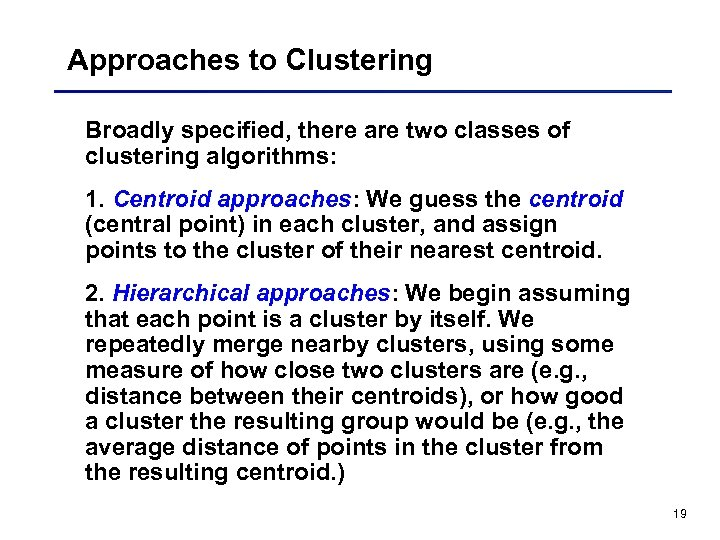Approaches to Clustering Broadly specified, there are two classes of clustering algorithms: 1. Centroid approaches: We guess the centroid (central point) in each cluster, and assign points to the cluster of their nearest centroid. 2. Hierarchical approaches: We begin assuming that each point is a cluster by itself. We repeatedly merge nearby clusters, using some measure of how close two clusters are (e. g. , distance between their centroids), or how good a cluster the resulting group would be (e. g. , the average distance of points in the cluster from the resulting centroid. ) 19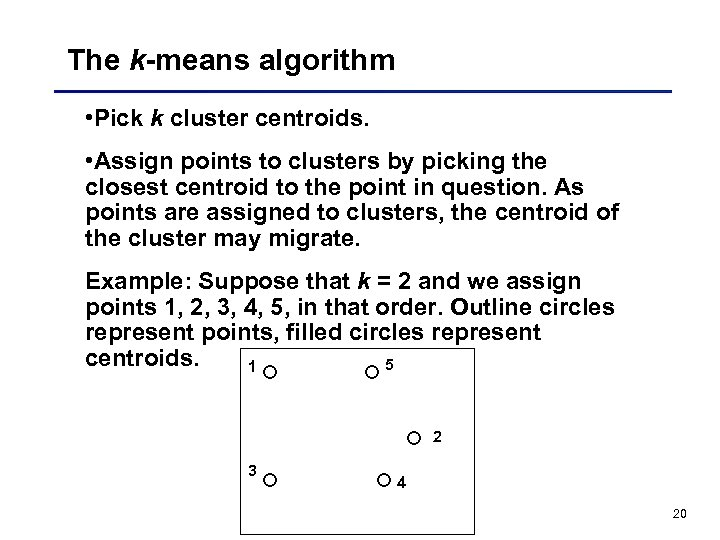The k-means algorithm • Pick k cluster centroids. • Assign points to clusters by picking the closest centroid to the point in question. As points are assigned to clusters, the centroid of the cluster may migrate. Example: Suppose that k = 2 and we assign points 1, 2, 3, 4, 5, in that order. Outline circles represent points, filled circles represent centroids. 5 1 2 3 4 20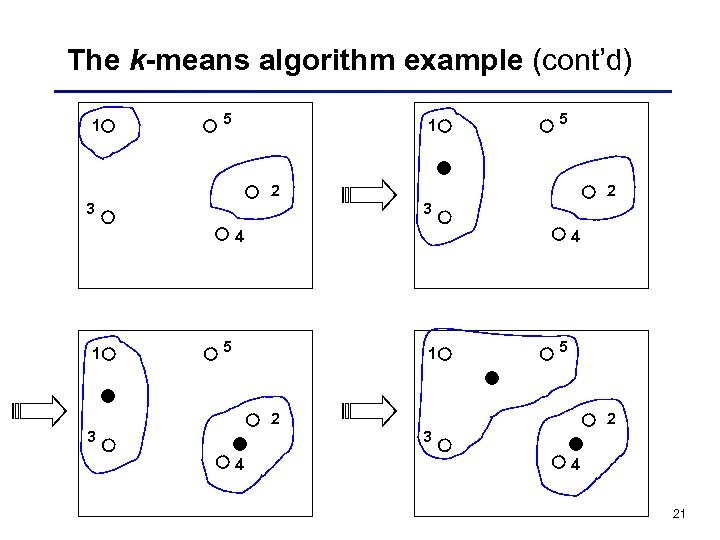The k-means algorithm example (cont’d) 1 5 2 3 4 1 4 5 1 5 2 3 4 4 21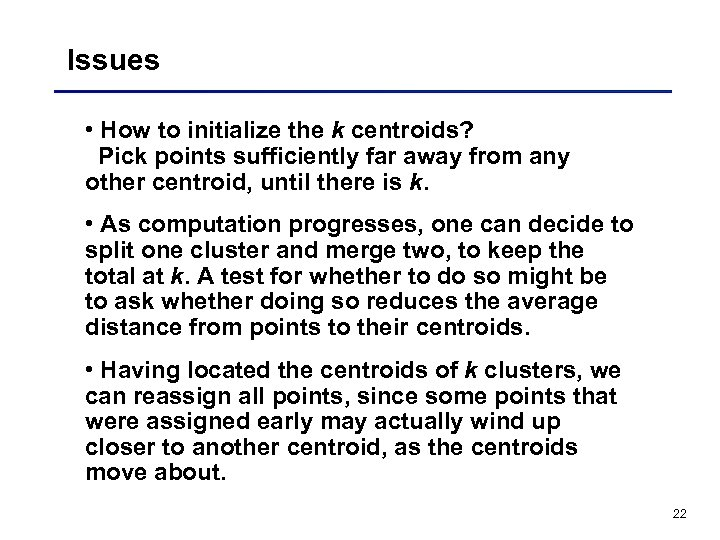Issues • How to initialize the k centroids? Pick points sufficiently far away from any other centroid, until there is k. • As computation progresses, one can decide to split one cluster and merge two, to keep the total at k. A test for whether to do so might be to ask whether doing so reduces the average distance from points to their centroids. • Having located the centroids of k clusters, we can reassign all points, since some points that were assigned early may actually wind up closer to another centroid, as the centroids move about. 22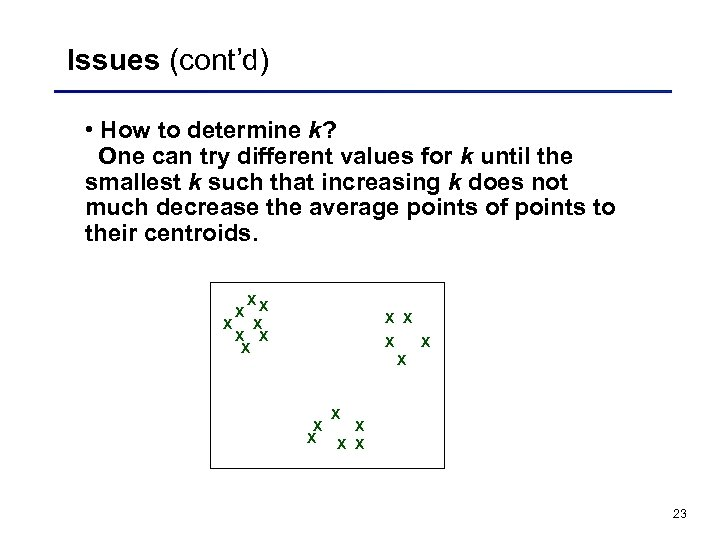Issues (cont’d) • How to determine k? One can try different values for k until the smallest k such that increasing k does not much decrease the average points of points to their centroids. X X X X X 23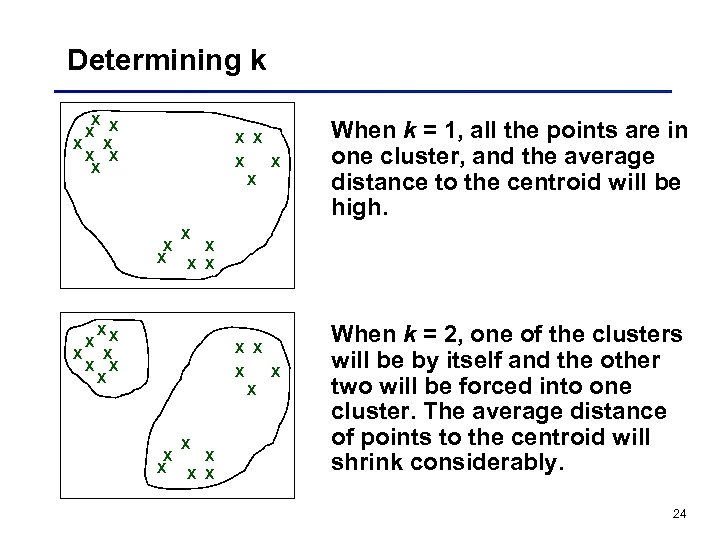Determining k X X X X X XX X X When k = 1, all the points are in one cluster, and the average distance to the centroid will be high. X X When k = 2, one of the clusters will be by itself and the other two will be forced into one cluster. The average distance of points to the centroid will shrink considerably. 24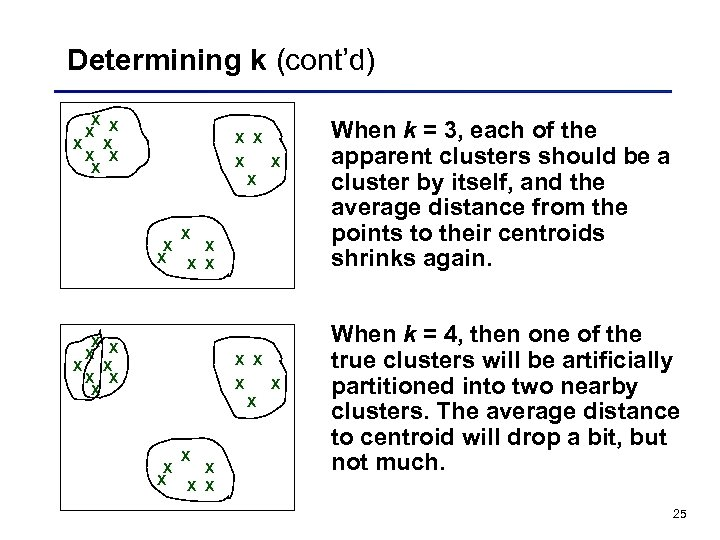Determining k (cont’d) X X X X X X X X X X When k = 3, each of the apparent clusters should be a cluster by itself, and the average distance from the points to their centroids shrinks again. When k = 4, then one of the true clusters will be artificially partitioned into two nearby clusters. The average distance to centroid will drop a bit, but not much. 25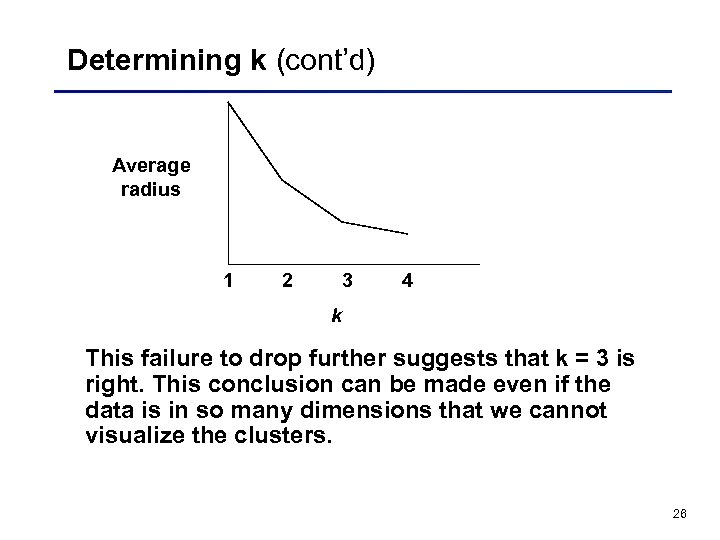Determining k (cont’d) Average radius 1 2 3 4 k This failure to drop further suggests that k = 3 is right. This conclusion can be made even if the data is in so many dimensions that we cannot visualize the clusters. 26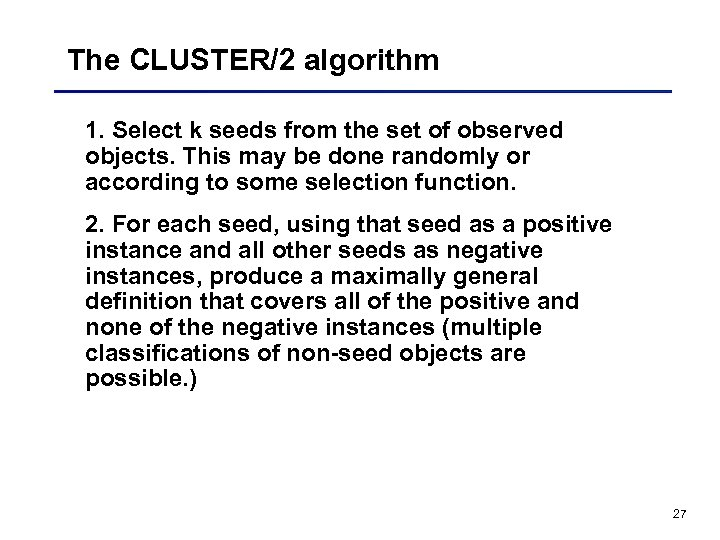The CLUSTER/2 algorithm 1. Select k seeds from the set of observed objects. This may be done randomly or according to some selection function. 2. For each seed, using that seed as a positive instance and all other seeds as negative instances, produce a maximally general definition that covers all of the positive and none of the negative instances (multiple classifications of non-seed objects are possible. ) 27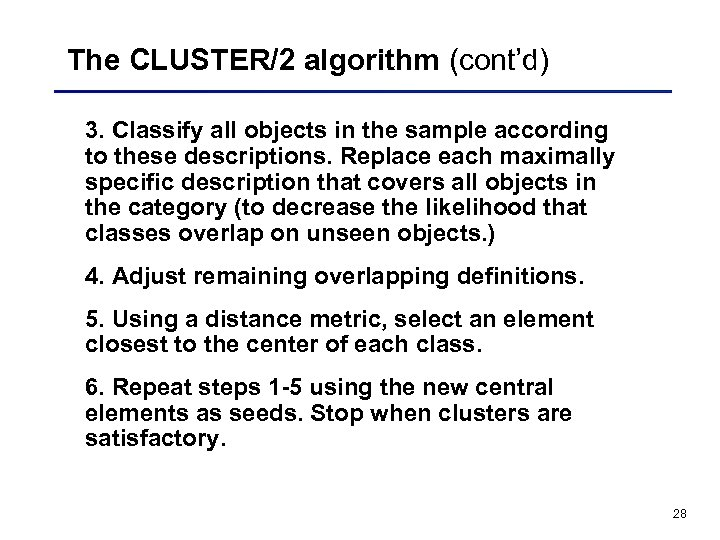The CLUSTER/2 algorithm (cont’d) 3. Classify all objects in the sample according to these descriptions. Replace each maximally specific description that covers all objects in the category (to decrease the likelihood that classes overlap on unseen objects. ) 4. Adjust remaining overlapping definitions. 5. Using a distance metric, select an element closest to the center of each class. 6. Repeat steps 1 -5 using the new central elements as seeds. Stop when clusters are satisfactory. 28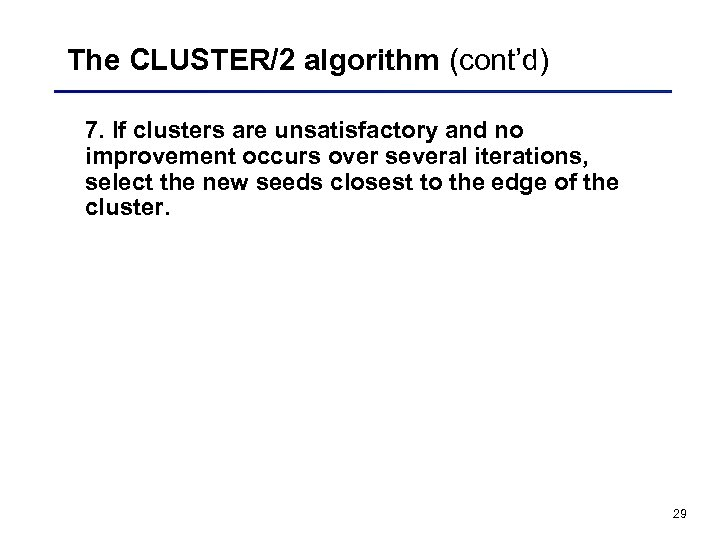The CLUSTER/2 algorithm (cont’d) 7. If clusters are unsatisfactory and no improvement occurs over several iterations, select the new seeds closest to the edge of the cluster. 29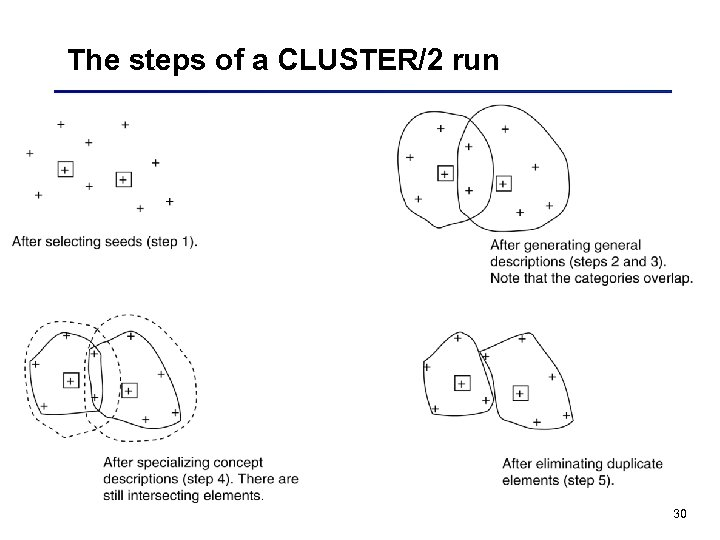The steps of a CLUSTER/2 run 30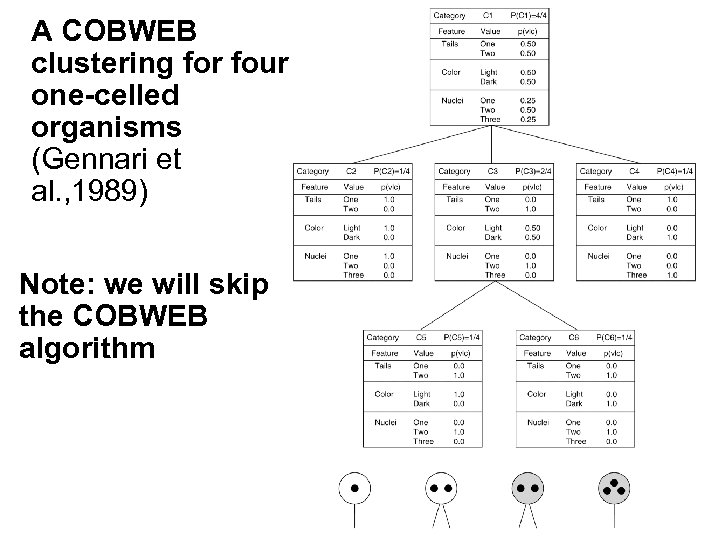A COBWEB clustering for four one-celled organisms (Gennari et al. , 1989) Note: we will skip the COBWEB algorithm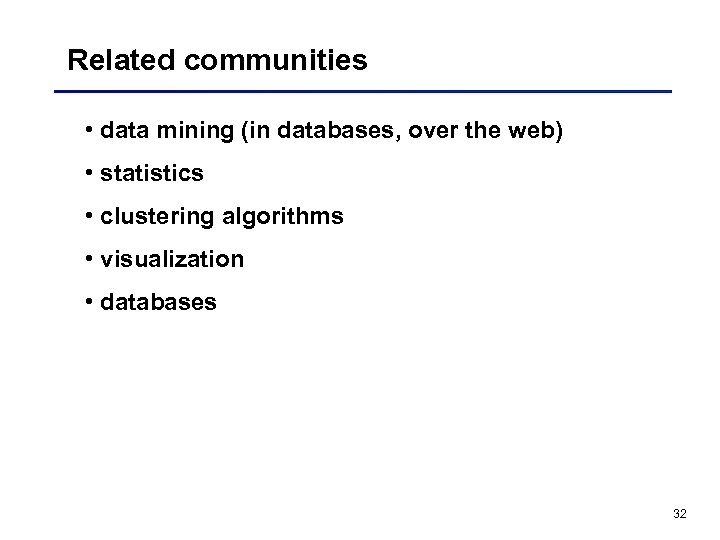Related communities • data mining (in databases, over the web) • statistics • clustering algorithms • visualization • databases 32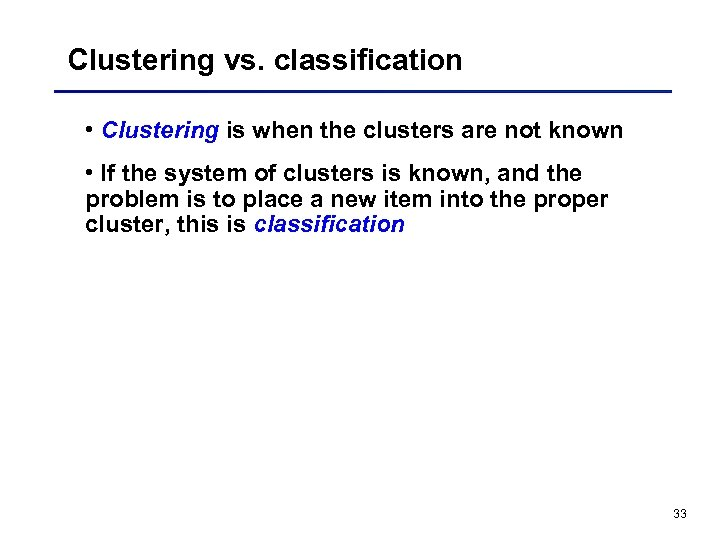Clustering vs. classification • Clustering is when the clusters are not known • If the system of clusters is known, and the problem is to place a new item into the proper cluster, this is classification 33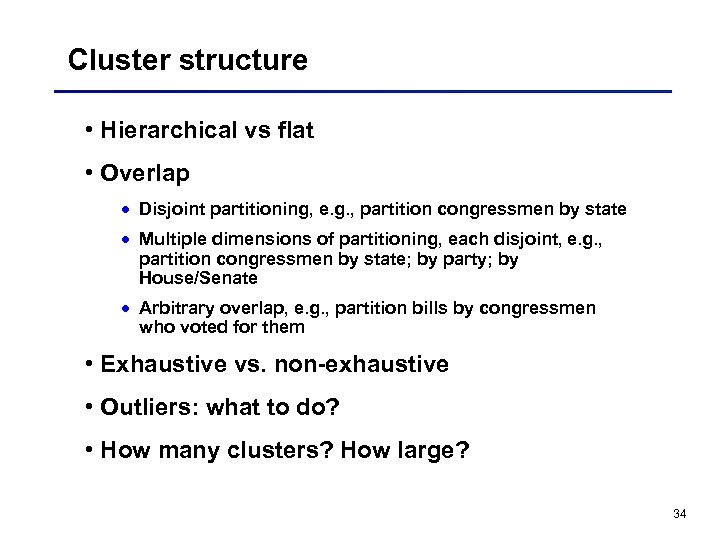Cluster structure • Hierarchical vs flat • Overlap · Disjoint partitioning, e. g. , partition congressmen by state · Multiple dimensions of partitioning, each disjoint, e. g. , partition congressmen by state; by party; by House/Senate · Arbitrary overlap, e. g. , partition bills by congressmen who voted for them • Exhaustive vs. non-exhaustive • Outliers: what to do? • How many clusters? How large? 34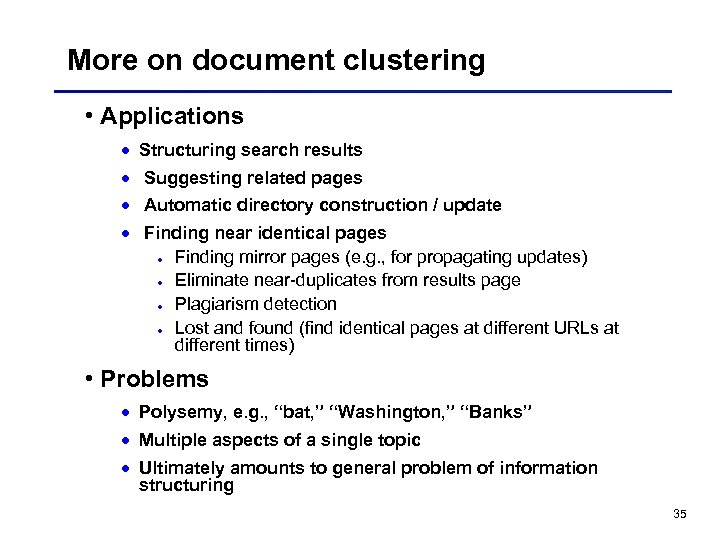More on document clustering • Applications · Structuring search results · Suggesting related pages · Automatic directory construction / update · Finding near identical pages · Finding mirror pages (e. g. , for propagating updates) · Eliminate near-duplicates from results page · Plagiarism detection · Lost and found (find identical pages at different URLs at different times) • Problems · Polysemy, e. g. , “bat, ” “Washington, ” “Banks” · Multiple aspects of a single topic · Ultimately amounts to general problem of information structuring 35